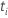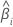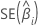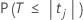# Methods and formulas for coefficients in Fit General Linear Model

Select the method or formula of your choice.

## Coefficient (Coef)

In matrix terms, the formula that calculates the vector of coefficients in the model is:

TermDescription
Xdesign matrix
Yresponse vector

## Standard error of the coefficient (SE Coef)

The standard errors of the coefficients for multiple regression are the square roots of the diagonal elements of this matrix:

### Notation

TermDescription
Xdesign matrix
X'transpose of the design matrix
s2mean square error

## T-value

### Notation

TermDescriptiontest statistic for thecoefficientestimated coefficientstandard error of theestimated coefficient

## P-value – Coefficients table

The two-sided p-value for the null hypothesis that a regression coefficient equals 0 is:

The degrees of freedom are the degrees of freedom for error, as follows:

np – 1

### Notation

TermDescriptionThe cumulative distribution function of the t distribution with degrees of freedom equal to the degrees of freedom for error.
tjThe t statistic for the jth coefficient.
nThe number of observations in the data set.
pThe sum of the degrees of freedom for the terms. The terms do not include the constant.